• 1.多层前馈神经网络 首先说下多层前馈神经网络，BP算法，BP神经网络之间的关系。多层前馈（multilayer feed-forward）神经网络由一个输入层、一个或多个隐藏层和一个输出层组成，后向传播(BP)算法在多层前馈神经...
一.多层前馈神经网络
首先说下多层前馈神经网络，BP算法，BP神经网络之间的关系。多层前馈[multilayer feed-forward]神经网络由一个输入层、一个或多个隐藏层和一个输出层组成，后向传播[BP]算法在多层前馈神经网络上面进行学习，采用BP算法的[多层]前馈神经网络被称为BP神经网络。给出一个多层前馈神经网络的拓扑结构，如下所示：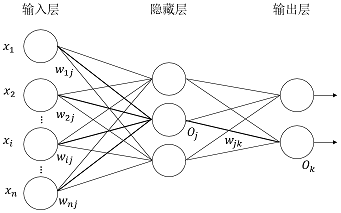神经网络的拓扑结构包括：输入层的单元数、隐藏层数[如果多于一层]、每个隐藏层的单元数和输出层的单元数。神经网络可以用于分类[预测给定元组的类标号]和数值预测[预测连续值输出]等。
二.后向传播[BP]算法详解
1.初始值权重
神经网络的权重被初始化为小随机数，每个神经元都有一个相关联的偏置，同样也被初始化为小随机数。
2.前向传播输入
以单个神经网络单元为例，如下所示：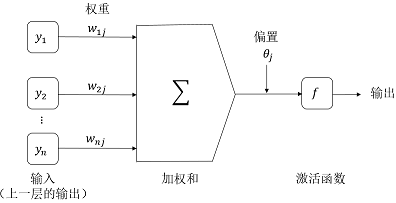给定隐藏层或输出层的单元jj，到单元jj的净输入IjI_{j}，如下所示：Ij=∑iwijOi+θj
{I_j} = \sum\limits_i {{w_{ij}}{O_i} + {\theta _j}}

其中，wijw_{ij}是由上一层的单元ii到单元jj的连接的权重；OiO_i是上一层的单元ii的输出；θj\theta_j是单元jj的偏置。需要说明的是偏置充当阀值，用来改变单元的活性。
给定单元jj的净输入IjI_j，单元jj的输出OjO_j，如下所示：Oj=11+e−Ij{O_j} = \frac{1}{{1 + {e^{ - {I_j}}}}}
3.后向传播误差
对于输出层单元jj，误差ErrjEr{r_j}用下式计算：Errj=Oj(1−Oj)(Tj−Oj)Er{r_j} = {O_j}\left( {1 - {O_j}} \right)\left( {{T_j} - {O_j}} \right)
其中，OjO_j是单元jj的实际输出，而TjT_j是jj给定训练元组的已知目标值。需要说明的是，Oj(1−Oj){O_j}\left( {1 - {O_j}} \right)是逻辑斯缔函数的导数。
对于隐藏层单元jj，它的误差用下式计算：Errj=Oj(1−Oj)∑kErrkwjkEr{r_j} = {O_j}\left( {1 - {O_j}} \right)\sum\limits_k {Er{r_k}{w_{jk}}}
其中，wjkw_{jk}是由下一较高层中单元kk到单元jj的连接权重，而ErrjEr{r_j}是单元kk的误差。
权重更新，如下所示：Δwij=(l)ErrjOiwij=wij+Δwij\begin{array}{l}
\Delta {w_{ij}} = \left( l \right)Er{r_j}{O_i} \\
{w_{ij}} = {w_{ij}} + \Delta {w_{ij}} \\
\end{array}
其中，Δwij\Delta {w_{ij}}是权重wijw_{ij}的改变量，变量ll是学习率，通常取0.0和1.0之间的常数值。
偏置更新，如下所示：Δθj=(l)Errjθj=θj+Δθj\begin{array}{l}
\Delta {\theta _j} = \left( l \right)Er{r_j} \\
{\theta _j} = {\theta _j} + \Delta {\theta _j} \\
\end{array}
其中，Δθj\Delta {\theta _j}是θj\theta_j的改变量。
权重和偏置更新
如果每处理一个样本就更新权重和偏置，称为实例更新[case update]；如果处理完训练集中的所有元组之后再更新权重和偏置，称为周期更新[epoch update]。理论上，反向传播算法的数据推导使用周期更新，但是在实践中，实例更新通常产生更加准确的结果。
说明：误差反向传播的过程就是将误差分摊给各层所有单元，从而获得各层单元的误差信号，进而修正各单元的权值，即权值调整的过程。
4.终止条件
如果满足条件之一，就可以停止训练，如下所示：
前一周期所有的都太小，小于某个指定的阀值。
前一周期误分类的元组百分比小于某个阀值。
超过预先指定的周期数。
实践中，权重收敛可能需要数十万个周期。神经网络的训练有很多的经验和技巧，比如可以使用一种称为模拟退火的技术，使神经网络确保收敛到全局最优。
三.用BP训练多层前馈神经网络
举个例子具体说明使用BP算法训练多层前馈神经网络的每个细节，如下所示：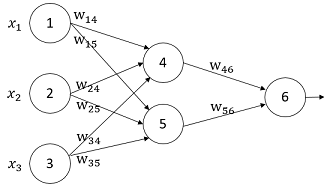设置学习率为0.9，第一个训练元组为X={1,0,1}X = \left\{ {1,0,1} \right\}，其类标号为1。神经网络的初始权重和偏置值如表1所示：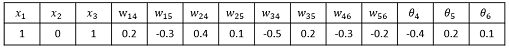根据给定的元组，计算每个神经元的净输入和输出，如表2所示：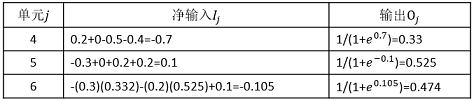每个神经元的误差值如表3所示：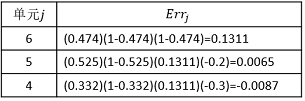说明：从误差的计算过程来理解反向[BP]传播算法也许更加直观和容易。
权重和偏置的更新如表4所示：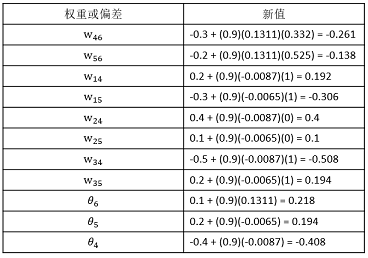说明：将该神经网络模型训练好后，就可以得到权重和偏执参数，进而做二分类。
四.用Python实现BP神经网络
神经网络拓扑结构，如下所示：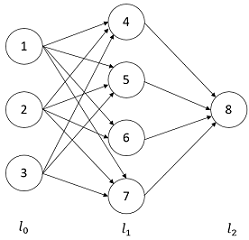解析：
1.第33和35行：l1和l2分别表示第1层和第2层神经元的输出。[第0层表示元组输入]
2.第37行：l2_error与相对应。
3.第40行：l2_delta与输出层误差相对应。
4.第42行：l1_error与相对应。
5.第43行：l1_delta与隐藏层误差相对应。
6.第45行：l1.T.dot(l2_delta)与相对应，而syn1与相对应。
7.第46行：l0.T.dot(l1_delta)与相对应，而syn0与相对应。
说明：
一边代码，一边方程，做到代码与方程的映射。这是一个基础的三层BP神经网络，但是麻雀虽小五脏俱全。主要的不足有几点：没有考虑偏置；没有考虑学习率；没有考虑正则化；使用的是周期更新，而不是实例更新[一个样本]和批量更新[m个样本]。但是，足以理解前馈神经网络和BP算法的工作原理。神经网络和BP算法的详细数学推导参考。
参考文献：
数据挖掘：概念与技术[第三版]
使用Python构造神经网络：http://www.ibm.com/developerworks/cn/linux/l-neurnet/
一个11行Python代码实现的神经网络：http://python.jobbole.com/82758/
用BP人工神经网络识别手写数字：http://blog.csdn.net/gzlaiyonghao/article/details/7109898
反向传导算法：http://ufldl.stanford.edu/wiki/index.php/%E5%8F%8D%E5%90%91%E4%BC%A0%E5%AF%BC%E7%AE%97%E6%B3%95


展开全文weight
• 提出了有理式多层前馈神经网络的数学模型, 给出了有理式多层神经网络的学习算法. 就计算复杂度而 言, 有理式神经网络的学习算法与传统的多层神经网络反传播算法是同阶的. 函数逼近应用实例结果表明, 将有理式...
•研究论文
• 多层前馈神经网络有哪些参数Machine learning is solving such a large number of sophisticated problems today that it seems like magic. But there isn’t any magic in machine learning rather it has a ...
多层前馈神经网络有哪些参数Machine learning is solving such a large number of sophisticated problems today that it seems like magic. But there isn’t any magic in machine learning rather it has a strong mathematical and statistical foundation.如今，机器学习正在解决如此众多的复杂问题，似乎就像魔术一样。 但是机器学习没有任何魔力，它具有强大的数学和统计基础。
While trying to understand the important and somewhat difficult concepts of machine learning, we sometimes do not even think about some of the trivial concepts. Maybe you think of those, but I know that I ignore a lot of simple things many a times. The reason is the amazing machine learning and deep learning libraries that have functions and methods to quickly do this for us. 😍 在尝试理解机器学习的重要且有些困难的概念时，我们有时甚至没有考虑一些琐碎的概念。 也许您会想到这些，但是我知道我很多次都忽略了许多简单的事情。 原因是令人惊叹的机器学习和深度学习库，它们具有可为我们快速完成此操作的功能和方法。 😍
One such trivial problem is to find the total number of trainable parameters in a feed-forward neural network by hand. A question that I encountered in one of my exams and confused me with the options provided. This question has also been asked in many different forums by many machine learnimg practitioners. 🙋🏻 一个这样的琐碎问题是用手找到前馈神经网络中可训练参数的总数。 我在一项考试中遇到的一个问题，使我对所提供的选项感到困惑。 许多机器学习实践者也在许多不同的论坛中提出了这个问题。 🙋🏻
The problem discussed in this post is: 这篇文章中讨论的问题是：
如何找到前馈神经网络中可训练参数的总数？
(How to find the total number of trainable parameters in a feed-forward neural network?)
You must be wondering why is that even an important one to discuss. It is indeed! The time taken to train a model is dependent on the number of parameters to train so this knowledge can really help us at times. 您一定想知道为什么这甚至是一个重要的话题。 这的确是！ 训练模型所需的时间取决于训练参数的数量，因此这些知识有时确实可以为我们提供帮助。
By looking at a simple network, you can easily count and tell the number of parameters. In the worst case, you can draw the diagram and tell the number of parameters. But what happens when you encounter a question of a neural network with 7 layers and a different number of neurons in each layer, say 8, 10, 12, 15, 15, 12, 6. How would you tell how many parameters are there in all? 通过查看一个简单的网络，您可以轻松地计算并告诉参数数量。 在最坏的情况下，您可以绘制图表并告诉参数数量。 但是，当您遇到一个神经网络的问题，该问题具有7层并且每层中有不同数量的神经元时，比如说8、10、12、15、15、12、6，该怎么办？所有？
Let us together find a mathematical formula to get the count. But before moving to the calculation, let us first understand what a feed-forward neural network is and what characteristics it possesses. This will help us in finding the total number of parameters. 让我们一起找到一个数学公式来获取计数。 但是在进行计算之前，让我们首先了解什么是前馈神经网络以及它具有的特征。 这将帮助我们找到参数的总数。
A feed-forward neural network is the simplest type of artificial neural network where the connections between the perceptrons do not form a cycle. 前馈神经网络是最简单的人工神经网络，其中感知器之间的连接不形成循环。Despite being the simplest neural network, they are of extreme importance to the machine learning practitioners as they form the basis of many important and advanced applications used today. 🤘 尽管它们是最简单的神经网络，但是它们对于机器学习从业者来说却极为重要，因为它们构成了当今使用的许多重要和高级应用程序的基础。 🤘
Characteristics of a feed-forward neural network: 前馈神经网络的特征：
Perceptrons are arranged in layers. The first layer takes in the input and the last layer gives the output. The middle layers are termed as hidden layers as they remain hidden from the external world. 感知器分层排列。 第一层接受输入，最后一层提供输出。 中间层被称为隐藏层，因为它们对外部世界保持隐藏。
Each perceptron in a layer is connected to each and every perceptron of the next layer. This is the reason for information flowing constantly from a layer to the next layer and hence the name feed-forward neural network. 一层中的每个感知器都连接到下一层的每个感知器。 这就是信息不断从一层流到下一层的原因，因此也就称为前馈神经网络。
There is no connection between the perceptrons of the same layer. 同一层的感知器之间没有连接。
There is no backward connection (called a feedback connection) from the current layer to the previous layer. 从当前层到上一层没有反向连接(称为反馈连接)。
Note: A perceptron is the fundamental unit of a neural network that calculates the weighted sum of the input values. 注意：感知器是计算输入值的加权和的神经网络的基本单位。
Mathematically, a feed-forward neural network defines a mapping y = f(x; θ) and learns the value of the parameters θ that helps in finding the best function approximation. 在数学上，前馈神经网络定义了一个映射y = f(x;θ)并学习了有助于寻找最佳函数逼近的参数θ的值。
Note: There is also a bias unit in a feed-forward neural network in all the layers except the output layer. Biases are extremely helpful in successful learning by shifting the activation function to the left or to the right. Confused? 🤔 In simple words, bias is similar to an intercept (constant) in a linear equation of the line, y = mx + c, which helps to fit the prediction line to the data better instead of a line always passing through the origin (0,0) (in case of y = mx). 注意：前馈神经网络中除输出层以外的所有层中还存在一个偏置单元。 偏向通过将激活功能向左或向右移动，对于成功学习非常有帮助。 困惑？ simple简单来说，偏差类似于直线的线性方程y = mx + c中的截距(常数)，这有助于使预测线更适合数据，而不是始终穿过原点的线(0 ，0)(如果y = mx)。
Let us now use this knowledge to find the number of parameters. 现在让我们使用此知识来查找参数的数量。
Scenario 1: A feed-forward neural network with just one hidden layer. Number of units in the input, hidden and output layers are respectively 3, 4 and 2. 方案1：仅具有一个隐藏层的前馈神经网络。 输入，隐藏和输出层中的单位数分别为3、4和2。
i = number of neurons in input layer i =输入层中神经元的数量
h = number of neurons in hidden layerh =隐藏层中神经元的数量
o = number of neurons in output layero =输出层中神经元的数量
From the diagram, we have i = 3, h = 4 and o = 2. Note that the red colored neuron is the bias for that layer. Each bias of a layer is connected to all the neurons in the next layer except the bias of the next layer.从图中，我们有i = 3， h = 4和o =2。请注意，红色神经元是该层的偏差。 一层的每个偏置都连接到下一层的所有神经元，除了下一层的偏置。
Mathematically: 数学上：
Number of connections between the first and second layer: 3 × 4 = 12, which is nothing but the product of i and h. 第一和第二层之间的连接数：3×4 = 12，这不过是i和h的乘积。Number of connections between the second and third layer: 4 × 2 = 8, which is nothing but the product of h and o. 第二层和第三层之间的连接数：4×2 = 8，这不过是h和o的乘积。There are connections between layers via bias as well. Number of connections between the bias of the first layer and the neurons of the second layer (except bias of the second layer): 1 × 4, which is nothing but h. 层之间也通过偏置连接。 第一层的偏置与第二层的神经元之间的连接数(第二层的偏置除外)：1×4，仅是h 。Number of connections between the bias of the second layer and the neurons of the third layer: 1 × 2, which is nothing but o. 第二层的偏置与第三层的神经元之间的连接数：1×2，不过是o 。Summing up all: 总结所有：
3 × 4 + 4 × 2 + 1 × 4 + 1 × 2 3×4 + 4×2 +1×4 +1×2
= 12 + 8 + 4 + 2 = 12 + 8 + 4 + 2
= 26 = 26
Thus, this feed-forward neural network has 26 connections in all and thus will have 26 trainable parameters. 因此，该前馈神经网络总共具有26个连接，因此将具有26个可训练的参数。
Let us try to generalize using this equation and find a formula. 让我们尝试使用该方程式进行概括并找到一个公式。
3 × 4 + 4 × 2 + 1 × 4 + 1 × 2 3×4 + 4×2 +1×4 +1×2
= 3 × 4 + 4 × 2 + 4 + 2 = 3×4 + 4×2 + 4 + 2
= i × h + h × o + h + o = i × h + h × o + h + o
Thus, the total number of parameters in a feed-forward neural network with one hidden layer is given by: 因此，具有一个隐藏层的前馈神经网络中的参数总数由下式给出：
(i × h + h × o) + h + o (i×h + h×o)+ h + o
Since this network is a small network it was also possible to count the connections in the diagram to find the total number. But, what if the number of layers is more? Let us work on one more scenario and see if this formula works or we need an extension to this. 由于该网络是小型网络，因此还可以对图中的连接进行计数以找到总数。 但是，如果层数更多怎么办？ 让我们在另一个场景中工作，看看这个公式是否有效，或者我们需要对此进行扩展。
Scenario 1: A feed-forward neural network with three hidden layers. Number of units in the input, first hidden, second hidden, third hidden and output layers are respectively 3, 5, 6, 4 and 2. 方案1：具有三个隐藏层的前馈神经网络。 输入，第一隐藏，第二隐藏，第三隐藏和输出层中的单元数分别为3、5、6、4和2。
Assumptions: 假设：
i = number of neurons in input layer i =输入层中神经元的数量
h1 = number of neurons in first hidden layerh1 =第一隐藏层中的神经元数量
h2 = number of neurons in second hidden layerh2 =第二个隐藏层中的神经元数量
h3 = number of neurons in third hidden layerh3 =第三隐藏层中的神经元数量
o = number of neurons in output layero =输出层中神经元的数量
Number of connections between the first and second layer: 3 × 5 = 15, which is nothing but the product of i and h1.第一层和第二层之间的连接数：3×5 = 15，这不过是i和h1的乘积。Number of connections between the second and third layer: 5 × 6 = 30, which is nothing but the product of h1 and h2. 第二层和第三层之间的连接数：5×6 = 30，仅是h1和h2的乘积。Number of connections between the third and fourth layer: 6 × 4 = 24, which is nothing but the product of h2 and h3. 第三层和第四层之间的连接数：6×4 = 24，这不过是h2和h3的乘积。Number of connections between the fourth and fifth layer: 4 × 2= 8, which is nothing but the product of h3 and o. 第四层与第五层之间的连接数：4×2 = 8，这不过是h3与o的乘积。Number of connections between the bias of the first layer and the neurons of the second layer (except bias of the second layer): 1 × 5 = 5, which is nothing but h1. 第一层的偏置与第二层的神经元之间的连接数(第二层的偏置除外)：1×5 = 5，仅是h1 。Number of connections between the bias of the second layer and the neurons of the third layer: 1 × 6 = 6, which is nothing but h2. 第二层的偏置与第三层的神经元之间的连接数：1×6 = 6，这不过是h2 。Number of connections between the bias of the third layer and the neurons of the fourth layer: 1 × 4 = 4, which is nothing but h3. 第三层的偏置与第四层的神经元之间的连接数：1×4 = 4，这不过是h3 。Number of connections between the bias of the fourth layer and the neurons of the fifth layer: 1 × 2 = 2, which is nothing but o. 第四层的偏置与第五层的神经元之间的连接数：1×2 = 2，这不过是o 。Summing up all: 总结所有：
3 × 5 + 5 × 6 + 6 × 4 + 4 × 2 + 1 × 5 + 1 × 6 + 1 × 4 + 1 × 2 3×5 + 5×6 + 6×4 + 4×2 +1×5 +1×6 +1×4 +1×2
= 15 + 30 + 24 + 8 + 5 + 6 + 4 + 2 = 15 + 30 + 24 + 8 + 5 + 6 + 4 + 2
= 94 = 94
Thus, this feed-forward neural network has 94 connections in all and thus 94 trainable parameters. 因此，该前馈神经网络总共具有94个连接，因此具有94个可训练参数。
Let us try to generalize using this equation and find a formula. 让我们尝试使用该方程式进行概括并找到一个公式。
3 × 5 + 5 × 6 + 6 × 4 + 4 × 2 + 1 × 5 + 1 × 6 + 1 × 4 + 1 × 2 3×5 + 5×6 + 6×4 + 4×2 +1×5 +1×6 +1×4 +1×2
= 3 × 5 + 5 × 6 + 6 × 4 + 4 × 2 + 5 + 6 + 4 + 2 = 3×5 + 5×6 + 6×4 + 4×2 + 5 + 6 + 4 + 2
= i × h1 + h1 × h2 + h2 × h3+ h3 × o + h1 + h2 + h3+ o = i × h1 + h1 × h2 + h2 × h3 + h3 × o + h1 + h2 + h3 + o
Thus, the total number of parameters in a feed-forward neural network with three hidden layers is given by: 因此，具有三个隐藏层的前馈神经网络中的参数总数由下式给出：
(i × h1 + h1 × h2 + h2 × h3 + h3 × o) + h1 + h2 + h3+ o (i×h1 + h1×h2 + h2×h3 + h3×o)+ h1 + h2 + h3 + o
ŤHUS，公式找到可训练参数的总数在具有n个隐藏层的前馈神经网络由下式给出：
(Thus, the formula to find the total number of trainable parameters in a feed-forward neural network with n hidden layers is given by:)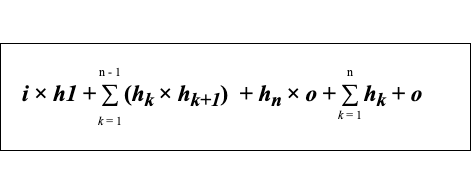Formula to find the total number of parameters in a feed-forward neural network (Image by author)If this formula sounds a bit overwhelming 😳, don’t worry, there is no need to memorize this formula 🙅. Just keep in mind that in order to find the total number of parameters we need to sum up the following: 如果这个公式听起来有点😳，不要担心，没有必要记住这个公式🙅。 请记住，为了找到参数总数，我们需要总结以下内容：
product of the number of neurons in the input layer and first hidden layer 输入层和第一个隐藏层中神经元数量的乘积
sum of products of the number of neurons between the two consecutive hidden layers两个连续的隐藏层之间的神经元数量的乘积之和product of the number of neurons in the last hidden layer and output layer最后一个隐藏层和输出层中神经元数量的乘积sum of the number of neurons in all the hidden layers and output layer所有隐藏层和输出层中神经元数量的总和Now, I hope you can apply this method to find the total number of parameters in a feed-forward neural network with any number of hidden layers and neurons even without using the library. 🙃现在，我希望您可以使用此方法来查找前馈神经网络中具有任意数量的隐藏层和神经元的参数总数，即使不使用该库也是如此。 🙃
Reference: 参考：
https://cs.stanford.edu/people/eroberts/courses/soco/projects/neural-networks/Architecture/feedforward.html https://cs.stanford.edu/people/eroberts/courses/soco/projects/neural-networks/Architecture/feedforward.html
Thank you! Do leave your comments if you have any feedback or suggestion regarding this post! 谢谢！ 如果您对此帖子有任何反馈或建议，请留下您的评论！
翻译自: https://towardsdatascience.com/number-of-parameters-in-a-feed-forward-neural-network-4e4e33a53655多层前馈神经网络有哪些参数
展开全文python java 深度学习 人工智能
• matlab开发-快速多层前馈神经网络训练。这是一个简单而快速的代码，可以训练任意层数的神经网络。
• 多层前馈神经网络善于解决非线性分析问题,但对于复杂的地质数据,普通的训练算法难以收敛。首先介绍了SPDS算法,并把它用于解决地质数据的非线性分析问题。算法的仿真实验表明,用SPDS算法训练的多层前馈神经网络,比较...
• 不同的层次结构会产出不同类型神经网络，比如前馈神经网络和递归神经网络。 神经元 神经元是神经网络最重要的组成部分，下图为一个神经元的示意图，箭头的方向就是数据运行的方向。为神经元接受的输入向量a，为...
神经网络-反向传播算法
神经网络-激活函数
神经网络是将许多个神经元按一定的层次结构连接起来的网络结构。不同的层次结构会产出不同类型神经网络，比如前馈神经网络和递归神经网络。

神经元

神经元是神经网络最重要的组成部分，下图为一个神经元的示意图，箭头的方向就是数据运行的方向。为神经元接受的输入向量a，为输入向量各个分量的权重w；b为偏置项bias，该条连接的输入固定为1。它可以让建模能力更强，更灵活。红色圆圈内为一个神经元处理信息的过程，里面又包括了SUM和f两部分。SUM为一个线性函数，将输入向量和权重向量的内积，输出的结果再传入激活函数f，最后输出结果t。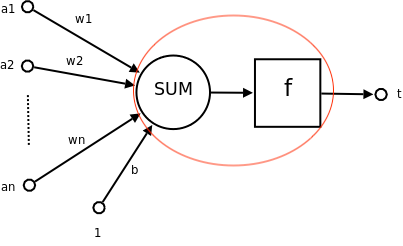一个神经元的功能是求得输入向量与权重向量的内积后，经过一个非线性函数也就是激活函数得到一个标量结果。

它会把一个n维向量空间用一个超平面分割成两部分，给定一个输入向量，神经元可以判断出这个向量位于超平面的哪一边。所以一个神经元就相当于做了一个二分类。(做回归任务的时候，神经元也相当于一个二分类吗？)

我们主要讲多层前馈神经网络。

多层前馈神经网络

多层前馈神经网络是由输入层，若干个隐藏层和输出层组成的全连接网络，不存在环或回路。。每一层都由多个神经元组成，神经元和输入向量全连接，但是神经元和神经元之间没有连接，也不存在跨层连接。每一层的输出结果会作为下一层的输入向量，比如hidden layer1的输出向量为hidden layer2的输入向量。输入层不会对数据进行处理，只是代表整个神经网络最开始的输入向量，也就是样本的特征向量。神经网络的输入为向量，最后的输出也是向量。输出向量的维度取决于输出层神经元的个数。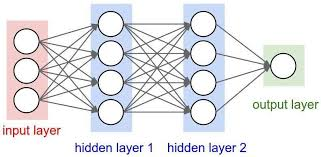多层前馈神经网络可以看作是非线性复合函数，它的学习过程就是将输入沿着网络结构的方向一层，一层的正向传播直到输出层，然后再通过反向传播算法 (BP) 对权重和偏置项进行跟更新。然后循环整个过程，直到满足最大迭代次数或者在验证集上的错误率变化不大。

当问题是回归问题时，损失函数为均方误差:

当问题是分类问题时，损失函数多为交叉熵，最后一层的激活函数为softmax函数:

, z为上一层神经元的输出

参考链接：https://zh.wikipedia.org/wiki/%E4%BA%BA%E5%B7%A5%E7%A5%9E%E7%BB%8F%E7%BD%91%E7%BB%9C

https://blog.csdn.net/qq_22690765/article/details/75050943

https://www.leiphone.com/news/201711/MWEDFvRMdOyN7Evm.html

展开全文神经网络
• 在这种结构中，将若干个单层神经网络级联在一起，前一层的输出作为后一层的输入，这样构成了多层前馈神经网络（ Multi-layer Feedforward Neural Networks ）。 更确切地说，每一层神经元仅与下一层的神经元全连接...
摘录自张玉宏的《深度学习之美》，这本书真的特别不错哦！
更一般的，常见的多层神经网络如图 8-2 所示。 在这种结构中，将若干个单层神经网络级联在一起，前一层的输出作为后一层的输入，这样构成了多层前馈神经网络（ Multi-layer Feedforward Neural Networks ）。 更确切地说，每一层神经元仅与下一层的神经元全连接。 但在同一层之内，神经元彼此不连接，而且跨层之间的神经元，彼此也不相连。
之所以加上 “前馈”这个定语，是想、特别强调，这样的网络是没有反馈的。 也就是说，位置靠后的层次不会把输出反向连接到之前的层次上作为输入，输入信号“一马平川”地单向向前传播。 很明显，相比于纵横交错的人类大脑神经元的连接结构，这种结构做了极大简化， 但即使如此，它也具有很强的表达力。
这种表达力强大到什么程度？奥地利学者库尔特 · 霍尼克（ Kurt Hornik ）等人的论文可以 旁证解释这个问题。 1989 年，霍尼克等人发表论文证明， 对于任意复杂度的连续波莱尔可测函数（ Borel Measurable Function ) f,，仅仅需要一个隐含层，只要这个隐含层包括足够多的神经元， 前馈神经网络使用挤压函数（ Squashing Function ）作为激活函数，就可以以任意精度来近似模拟$f$.
$f:R^N\rightarrow R^M$
如果想增加f的近似精度，单纯依靠增加神经元的数目即可实现。 换句话说，多层前馈神经网络可视为一个通用函数的模拟器（ Universal Approximators ）。 对于这个定理证明的可视化描述，读者可参阅迈克尔·尼尔森（ Michael Nielsen ）撰写的系列博客［5］(httP://neuralnetworksanddeeplearning.com/chap4.html)。 在他博客的第 4 章中， 尼尔森给出了神经网络可计算任何函数的可视化证明，值得一读。
然而， 如何确定隐含层的个数是一个超参数问题，即不能通过网络学习自行得到，而是需要人们通过试错法外加经验甚至直觉来调整。


展开全文• 提出了一种基于二元蚁群算法的多层前馈神经网络,同时为了避免二元蚁群算法陷入局部最优引入了拥挤交通组织策略。将二元蚁群算法和神经网络混合,可兼有神经网络广泛映射能力和二元蚁群算法快速全局收敛能力,通过在...
• BP神经网络多指多层前馈神经网络，运用梯度下降法，最小化误差。设神经网络最后输出l{l}l维向量为[y1,y2,....,yl][y_{1},y_{2},....,y_{l}][y1​,y2​,....,yl​],实际的输出为[y^1,y^2...,y^l][\hat{y}_1,\hat{y}_2...python 机器学习
• 此代码使用一阶随机梯度下降优化多层前馈神经网络。 它将网络输出为一个结构，然后可以在新数据上进行测试。matlab
• 前馈神经网络的图例及推导过程见https://blog.csdn.net/u010089444/article/details/52555567，接下来我们用python语言实现多层前馈神经网络。本例使用的是MINST数据集，由输入层,两个隐藏层,输出层. MNIST数据集中...Tensorflow 手写体识别
• 1.多层前馈神经网络 首先说下多层前馈神经网络，BP算法，BP神经网络之间的关系。多层前馈（multilayer feed-forward）神经网络由一个输入层、一个或多个隐藏层和一个输出层组成，后向传播(BP)算法在多层前馈神经...
• M-P神经元模型 神经网络中最基本的组成成分：神经元模型。如下图是一个典型的“M-P神经元模型”： 上图中，神经元接收到n个其他神经元传递过来的输入...多层前馈神经网络 定义：每层神经元与下一层神经元全互...BP算法推导 反向传播算法 误差逆传播算法 深度学习
• 一种多层前馈神经网络的快速修剪算法，对网络的修剪有所帮助。
• 构建一个至少含有1-2 层隐藏层的神经网络模型，解决手写的0-9 十个手写 数字的识别问题。神经网络模型构建过程中需要注意的几点： （1）数据集采用MNIST 阿拉伯数字手写体数据集。 （2）模型输入层的节点个数的设计...python
• 使用Matlab实现神经网络2.1 newff函数2.1.1 newff函数语法2.1.2 常用的激活函数2.1.3 常见的训练函数2.1.4 网络配置参数2.2 train函数2.3 sim函数3. 参数设置对神经网络性能的影响3.1 隐含层节点个数3.2 激活函数的...算法 Algorithm
• 针对目前神经网络在应用中难于确定隐层神经元数的问题,提出了一种神经网络结构的快速修剪算法。该 算法在最优脑外科算法( OBS)的基础上,通过直接剔除冗余的隐层神经元实现神经网络结构自组织设计。实验结果 表明,...
• 因为公式都是在mathtype上写的，然后插入到ppt的，而且里面有很多图，再用MD写一遍简直太耗时间，所以我就直接当作图片插入发博客了，还请读者谅解。本来ppt是我们每周开组会用的，相互讲解交流，自己做这些也废了...深度学习 神经网络
• TensorFlow是通过构建图来运行操作，对应于多层前馈神经网络，需要构筑2个隐藏层，mnist.inference就是这样的函数，其hidden_layer1是 tf.nn.relu(tf.matmul(images, weights) + biases)，用激活函数relu来运算 wx+b...tensorflow 深度学习
• 老师给的课件算错了== 验证了一下 1 #include<iostream>... 3 //前馈神经网络解决异或问题 4 using namespace std; 5 int sgn(float x){ 6 if(x<0) {return 0;} 7 else re...
• 单层感知机： ...从神经元细胞借鉴的‘门限’和‘权重和’的概念是建模的关键。 向量xw的点积--》海维赛德阶跃函数（作为激活函数）--》分类0，1 XW+b, b为偏好。如果b为负，样本X一定，为使结果仍为0.5，要激活函数 非线性模型
• 神经网络灵敏度分析对网络结构设计、硬件实现等具有重要的指导意义，已有的灵敏度计算公式对权值和输入扰动有一定限制或者计算误差较大。基于Piché的随机模型，通过使用两个逼近函数对神经网络一类Sigmoid激活函数......